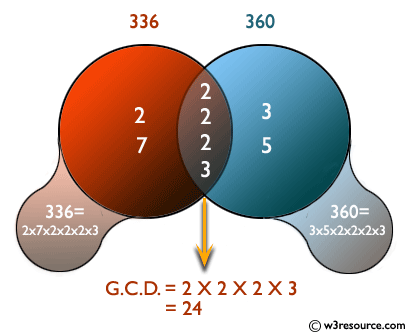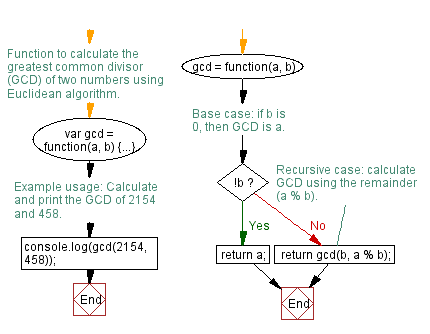# JavaScript: Find the greatest common divisor of two positive numbers

## JavaScript Function: Exercise-2 with Solution

Write a JavaScript program to find the greatest common divisor (gcd) of two positive numbers.

Pictorial Presentation:Sample Solution:-

HTML Code:

``````<!DOCTYPE html>
<html>
<meta charset="utf-8">
<title>Recursive function to find the GCD of two numbers</title>
<body>

</body>
</html>
```
```

JavaScript Code:

``````var gcd = function(a, b) {
if ( ! b) {
return a;
}

return gcd(b, a % b);
};
console.log(gcd(2154, 458));
```
```

Output:

```2
```

Flowchart:Live Demo:

See the Pen javascript-recursion-function-exercise-2 by w3resource (@w3resource) on CodePen.

Improve this sample solution and post your code through Disqus

What is the difficulty level of this exercise?

Test your Programming skills with w3resource's quiz.

﻿

## JavaScript: Tips of the Day

Returns the unique symmetric difference between two arrays, not containing duplicate values from either array

Example:

```const uniqueSymmetricDifference = (a, b) => [
...new Set([...a.filter(v => !b.includes(v)), ...b.filter(v => !a.includes(v))])
];
console.log(uniqueSymmetricDifference([1, 2, 3], [1, 2, 4])); // [3, 4]
console.log(uniqueSymmetricDifference([1, 2, 2], [1, 3, 1])); // [2, 3]
```

Output:

```[3, 4]
[2, 3]
```